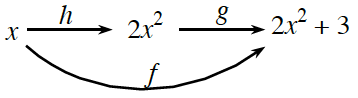### Home > CALC3RD > Chapter Ch5 > Lesson 5.1.4 > Problem5-44

5-44.

A “CHAIN” OF FUNCTIONS

Remember that composite functions are made by “chaining” simpler functions together. For example, $f\left(x\right) = 2x^{2} + 3$ is made by chaining the functions $h\left(x\right) = 2x^{2}$ and $g\left(x\right) = x + 3$.

It can be useful to show this idea with an arrow diagram:Above is an arrow diagram for $h$ followed by $g$, which is written $f(x) = g(h(x))$. (Notice that the “inner” function is applied first.)

Let $h(x) = \tan(x) + 5 \ \text {and}\ g(x) = (x + 3)^2$. Draw an arrow diagram and determine the composite function for each part below. Simplifying is not required.

1. $f(x) = g(h(x))$

$x\overset{h}{\rightarrow}\text{tan } x+5\overset{g}{\rightarrow}((\text{tan }x+5)+3)^{2}=(\text{tan }x+8)^{2}$

2. $j(x) = h(g(x))$

Refer to example above and hint in part (a).

3. $k(x) = g(g(h(x)))$

Refer to example above and hint in part (a).

4. $n(x) = h(g(g(x)))$

Refer to example above and hint in part (a).